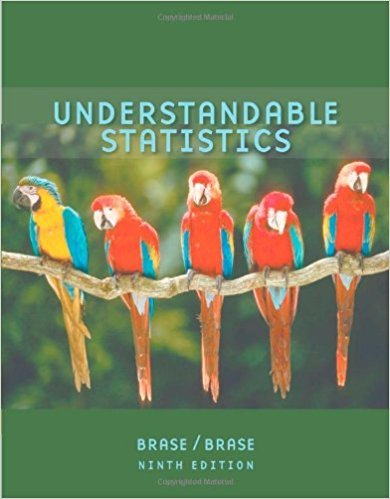×
×

# Solutions for Chapter 6.4: NORMAL DISTRIBUTIONS## Full solutions for Understandable Statistics | 9th Edition

ISBN: 9780618949922Solutions for Chapter 6.4: NORMAL DISTRIBUTIONS

Solutions for Chapter 6.4
4 5 0 335 Reviews
23
1
##### ISBN: 9780618949922

Since 14 problems in chapter 6.4: NORMAL DISTRIBUTIONS have been answered, more than 35868 students have viewed full step-by-step solutions from this chapter. This expansive textbook survival guide covers the following chapters and their solutions. Chapter 6.4: NORMAL DISTRIBUTIONS includes 14 full step-by-step solutions. Understandable Statistics was written by and is associated to the ISBN: 9780618949922. This textbook survival guide was created for the textbook: Understandable Statistics, edition: 9.

Key Statistics Terms and definitions covered in this textbook
• Acceptance region

In hypothesis testing, a region in the sample space of the test statistic such that if the test statistic falls within it, the null hypothesis cannot be rejected. This terminology is used because rejection of H0 is always a strong conclusion and acceptance of H0 is generally a weak conclusion

• Asymptotic relative eficiency (ARE)

Used to compare hypothesis tests. The ARE of one test relative to another is the limiting ratio of the sample sizes necessary to obtain identical error probabilities for the two procedures.

• Attribute

A qualitative characteristic of an item or unit, usually arising in quality control. For example, classifying production units as defective or nondefective results in attributes data.

• Chi-square (or chi-squared) random variable

A continuous random variable that results from the sum of squares of independent standard normal random variables. It is a special case of a gamma random variable.

• Continuous distribution

A probability distribution for a continuous random variable.

• Contour plot

A two-dimensional graphic used for a bivariate probability density function that displays curves for which the probability density function is constant.

• Convolution

A method to derive the probability density function of the sum of two independent random variables from an integral (or sum) of probability density (or mass) functions.

• Critical value(s)

The value of a statistic corresponding to a stated signiicance level as determined from the sampling distribution. For example, if PZ z PZ ( )( .) . ? =? = 0 025 . 1 96 0 025, then z0 025 . = 1 9. 6 is the critical value of z at the 0.025 level of signiicance. Crossed factors. Another name for factors that are arranged in a factorial experiment.

• Cumulative normal distribution function

The cumulative distribution of the standard normal distribution, often denoted as ?( ) x and tabulated in Appendix Table II.

• Decision interval

A parameter in a tabular CUSUM algorithm that is determined from a trade-off between false alarms and the detection of assignable causes.

• Degrees of freedom.

The number of independent comparisons that can be made among the elements of a sample. The term is analogous to the number of degrees of freedom for an object in a dynamic system, which is the number of independent coordinates required to determine the motion of the object.

• Deming

W. Edwards Deming (1900–1993) was a leader in the use of statistical quality control.

• Discrete distribution

A probability distribution for a discrete random variable

• Eficiency

A concept in parameter estimation that uses the variances of different estimators; essentially, an estimator is more eficient than another estimator if it has smaller variance. When estimators are biased, the concept requires modiication.

• Erlang random variable

A continuous random variable that is the sum of a ixed number of independent, exponential random variables.

• Error variance

The variance of an error term or component in a model.

• Estimate (or point estimate)

The numerical value of a point estimator.

• Expected value

The expected value of a random variable X is its long-term average or mean value. In the continuous case, the expected value of X is E X xf x dx ( ) = ?? ( ) ? ? where f ( ) x is the density function of the random variable X.

• Fraction defective control chart

See P chart

• Geometric mean.

The geometric mean of a set of n positive data values is the nth root of the product of the data values; that is, g x i n i n = ( ) = / w 1 1 .

×